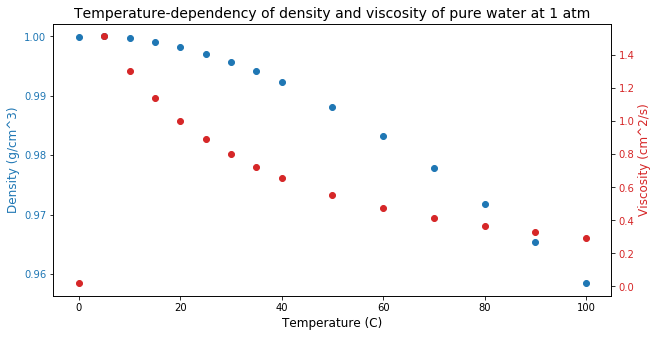# Reading the Data¶

A table with the variation of density and viscosity in terms of the temperature, at a fixed pressure of $1$ atmosphere, is available in Batchelor (2000). The data has been digitized and saved into a local csv file. Here we load the table from the file and view and plot the data.

## Importing the libraries¶

First we import the libraries used in this particular notebook.

In :
import pandas as pd
import matplotlib.pyplot as plt


## Using pandas¶

The data has been digitized to the local file water.csv. An easy way to retrieve it is with the pandas.read_csv() function of the pandas library:

In :
water_pd = pd.read_csv('water.csv', header=[0,1])


### Viewing the data with pandas¶

The data is diplayed nicely with pandas:

In :
water_pd

Out:
temp density viscosity
Temperature (C) Density (g/cm^3) Viscosity (cm^2/s)
0 0 0.9999 0.01787
1 5 1.0000 1.51400
2 10 0.9997 1.30400
3 15 0.9991 1.13800
4 20 0.9982 1.00400
5 25 0.9971 0.89400
6 30 0.9957 0.80200
7 35 0.9941 0.72500
8 40 0.9923 0.65900
9 50 0.9881 0.55400
10 60 0.9832 0.47500
11 70 0.9778 0.41400
12 80 0.9718 0.36600
13 90 0.9653 0.32700
14 100 0.9584 0.29500

### Plotting the data¶

We may also visualize both variations of density and viscosity using matplotlib.pyplot:

In :
fig, ax1 = plt.subplots(figsize=(10,5))

color = 'tab:blue'
ax1.set_xlabel(water_pd['temp'].columns, fontsize=12)
ax1.set_ylabel(water_pd['density'].columns, color=color, fontsize=12)
ax1.plot(water_pd['temp'], water_pd['density'], 'o', color=color)
ax1.tick_params(axis='y', labelcolor=color)

ax2 = ax1.twinx()

color = 'tab:red'
ax2.set_ylabel(water_pd['viscosity'].columns, color=color, fontsize=12)
ax2.plot(water_pd['temp'], water_pd['viscosity'], 'o', color=color)
ax2.tick_params(axis='y', labelcolor=color)

plt.title('Temperature-dependency of density and viscosity of pure water at 1 atm',
fontsize=14)
plt.show()